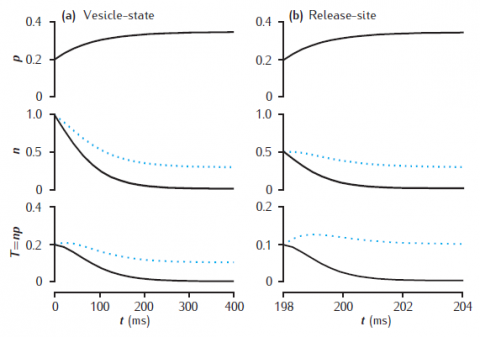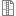# Figure 7.10Deterministic models of short-term synaptic dynamics

Facilitation and depression in deterministic models of short-term synaptic dynamics. Phenomenological model of facilitation: p0 = 0.2; Δp = 0.05; τf = 100 ms. (a) vesicle-state model: kn = kr = 0.2 /s. (b) release-site model: nT = 1; kn = kr = 0.2 /s. Solid lines: ns = 0; dotted lines: ns = 0.1. Synapse stimulated at 50Hz.

Simulation environment:
Notes

Unzip file and run runsynnp_rv.m in MATLAB.synnp_rv.zip